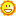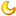## 量子网络

#### #楼主# 2020-9-19量子网络 开始 BB84 BBM92 E91 DPS 牛 DARPA量子网络 2001 是 没有 没有 没有 没有 维也纳的SECOCQ QKD网络 2003年 是 是 没有 没有 是 东京QKD网络 2009年 是 是 没有 是 没有 中国芜湖的层次网络 2009年 是 没有 没有 没有 没有 日内瓦局域网（SwissQuantum） 2010 是 没有 没有 没有 是

DARPA量子网络从2000年代初开始，DARPA开始赞助量子网络开发项目，旨在实施安全通信。在DARPA量子网络成为内的业务BBN技术公司在2003年底实验室于2004年进一步扩大到包括哈佛大学和波士顿大学的节点。该网络由多个物理层组成，包括支持相位调制激光器和纠缠光子的光纤以及自由空间链路。SECOQC维也纳QKD网络从2003年到2008年，基于量子密码学的安全通信（SECOQC）项目在许多欧洲机构之间建立了协作网络。SECOQC项目选择的体系结构是一种受信任的转发器体系结构，它由设备之间的点对点量子链接组成，这些设备之间通过使用转发器实现长距离通信。中文分级网络2009年5月，在中国芜湖展示了一个分层的量子网络。分层网络由连接四个子网的四个节点的骨干网组成。骨干节点通过光交换量子路由器连接。每个子网内的节点也通过光交换机连接，并通过受信任的中继连接到骨干网。日内瓦局域网（SwissQuantum）SwissQuantum网络在2009年至2011年之间开发并测试了欧洲核子研究中心与日内瓦大学和日内瓦Hepia相连的设施。SwissQuantum计划的重点是将SECOQC和其他研究量子网络中开发的技术过渡到生产环境。特别是与现有电信网络的集成及其可靠性和健壮性。东京QKD网络2010年，来自日本和欧盟的许多组织建立并测试了东京QKD网络。东京网络基于现有的QKD技术，并采用了类似SECOQC的网络体系结构。这是第一次，一次性垫加密在足够高的数据速率实现，以支持流行的最终用户的应用，如安全语音和视频会议。先前的大规模QKD网络通常使用经典的加密算法（例如AES）进行高速率数据传输，并使用量子派生的密钥来获取低速率数据或定期重新加密经典加密算法。京沪干线2017年9月，中国北京和上海之间的2000公里量子密钥分配网络正式开放。这条干线将充当连接北京，上海，山东济南和安徽合肥的量子网络的骨干网。在开幕式上，交通银行的两名员工使用该网络完成了从上海到北京的交易。中国国家电网公司也在开发该链接的管理应用程序。该行使用32个受信节点作为转发器。量子电信网络也已经在中国中部湖北省省会武汉市投入使用，该网络将与干线相连。计划沿长江沿线其他类似的城市量子网络。现代物理学 {\ displaystyle {\ hat {H}} | \ psi _ {n}（t）\ rangle = i \ hbar {\ frac {\ partial} {\ partial t}} || \ psi _ {n}（t）\ angle{\ displaystyle {\ frac {1} {{c} ^ {2}}}} {\ frac {{\ partial} ^ {2} {\ phi} _ {n}} {{\ partial t} ^ {2} }}-{{\ nabla} ^ {2} {\ phi} _ {n}} + {\ left（{\ frac {mc} {\ hbar}} \ right）} ^ {2} {\ phi} _ {n} = 0}{\ displaystyle E = h \ nu \}1927年在布鲁塞尔举行的索尔维会议是第五次世界物理学会议。

• 量化某些物理性质
• 量子纠缠
• 不确定性原理
• 波粒对偶• 经典系统的许多宏观特性是其零件的量子行为的直接结果。例如，大块物质的稳定性（由仅在电力作用下会迅速坍塌的原子和分子组成），固体的刚度以及物质的机械，热，化学，光学和磁性等都是相互作用的结果。量子力学规则下的电荷
• 尽管对于极小的粒子或接近光速的速度，由量子力学和相对论所提出的看似“异乎寻常”的物质行为变得更加明显，但通常被认为是“ 牛顿 ” 论的经典定律，物理学仍然可以准确地预测行为绝大多数“大”物体（大约是大分子的大小或更大）的运动速度远小于光速

1956年提出的埃弗里特多世界解释认为，量子理论描述的所有可能性同时发生在由独立的平行宇宙组成的多重宇宙中。这不是通过向量子力学引入“新公理”来实现的，而是通过消除波包坍塌的公理来实现的。被测系统和测量设备（包括观察者）的所有可能的一致状态都以真实的物理形式存在-不仅像其他解释中那样形式上是数学上的- 量子叠加。不同系统的一致状态组合的这种叠加称为纠缠状态。尽管多元宇宙是确定性的，但我们会感知到由概率控制的非确定性行为，因为我们只能观察到作为观察者所居住的宇宙（即，对上述叠加的一致状态贡献）。Everett的解释与John Bell的实验完全一致，并使它们直观易懂。但是，根据量子退相干理论，这些“平行宇宙”将是我们永远无法接近的。不可访问性可以理解为：一旦完成测量，被测系统就会与两个系统纠缠在一起。测量它的物理学家大量其他粒子，其中一些是光子，以光速飞向宇宙的另一端。为了证明波函数没有崩溃，必须将所有这些粒子带回并再次测量它们，以及最初测量的系统。这不仅是完全不切实际的，而且即使从理论上讲可以做到这一点，也必须破坏任何进行原始测量的证据（包括物理学家的记忆）。{\ displaystyle-{\ frac {\ hbar ^ {2}} {2m}} {\ frac {d ^ {2} \ psi} {dx ^ {2}}} = E \ psi。}{\ displaystyle {\ hat {p}} _ {x} =-i \ hbar {\ frac {d} {dx}}}{\ displaystyle {\ frac {1} {2m}} {\ hat {p}} _ {x} ^ {2} = E，}{\ displaystyle \ psi（x）= Ae ^ {ikx} + Be ^ {-ikx} \ qquad \ qquad E = {\ frac {\ hbar ^ {2} k ^ {2}} {2m}}}{\ displaystyle \ psi（x）= C \ sin（kx）+ D \ cos（kx）。\！}{\ displaystyle \ psi（0）= 0 = C \ sin（0）+ D \ cos（0）= D}{\ displaystyle \ psi（L）= 0 = C \ sin（kL），}{\ displaystyle k = {\ frac {n \ pi} {L}} \ qquad \ qquad n = 1,2,3，\ ldots。}{\ displaystyle E = {\ frac {\ hbar ^ {2} \ pi ^ {2} n ^ {2}} {2mL ^ {2}}} = {\ frac {n ^ {2} h ^ {2} } {8mL ^ {2}}}。}{\ displaystyle \ psi（x）= C \ sin（kx）+ D \ cos（kx）。}{\ displaystyle \ psi _ {n}（x）= {\ begin {cases} A \ cos（k_ {n} x），＆n = 1,3,5，\ dots \\ B \ sin（k_ {n} x），＆n = 2,4,6，\点\ end {cases}}}{\ displaystyle V（x）= {\ frac {1} {2}} m \ omega ^ {2} x ^ {2}。}{\ displaystyle \ psi _ {n}（x）= {\ sqrt {\ frac {1} {2 ^ {n} \，n！}}}} \ cdot \ left（{\ frac {m \ omega} {\ pi \ hbar}} \ right）^ {1/4} \ cdot e ^ {-\\ frac {m \ omega x ^ {2}} {2 \ hbar}}} \ cdot H_ {n} \ left（{ \ sqrt {\ frac {m \ omega} {\ hbar}}} x \ right），\ qquad}{\ displaystyle n = 0,1,2，\ ldots。}{\ displaystyle H_ {n}（x）=（-1）^ {n} e ^ {x ^ {2}} {\ frac {d ^ {n}} {dx ^ {n}}} \ left（e ^ {-x ^ {2}} \ right），}{\ displaystyle E_ {n} = \ hbar \ omega \ left（n + {1 \ over 2} \ right）。}<math xmlns="http://www.w3.org/1998/Math/MathML" alttext="{\displaystyle V(x)={\begin{cases}0,&x{\ displaystyle V（x）= {\ begin {cases} 0，＆x <0，\\ V_ {0}，＆x \ geq 0. \ end {cases}}}<math xmlns="http://www.w3.org/1998/Math/MathML" alttext="{\displaystyle \psi _{1}(x)={\frac {1}{\sqrt {k_{1}}}}\left(A_{\rightarrow }e^{ik_{1}x}+A_{\leftarrow }e^{-ik_{1}x}\right)\qquad x{\ displaystyle \ psi _ {1}（x）= {\ frac {1} {\ sqrt {k_ {1}}}}} \ left（A _ {\ rightarrow} e ^ {ik_ {1} x} + A_ { \ leftarrow} e ^ {-ik_ {1} x} \ right）\ qquad x <0}0}">{\ displaystyle \ psi _ {2}（x）= {\ frac {1} {\ sqrt {k_ {2}}}}} \ left（B _ {\ rightarrow} e ^ {ik_ {2} x} + B_ { \ leftarrow} e ^ {-ik_ {2} x} \ right）\ qquad x> 0}0}" style="border: 0px; vertical-align: -3.171ex; display: inline-block; width: 47.791ex; height: 6.509ex;">，

{\ displaystyle k_ {1} = {\ sqrt {2mE / \ hbar ^ {2}}}}{\ displaystyle k_ {2} = {\ sqrt {2m（E-V_ {0}）/ \ hbar ^ {2}}}}• 根据量子力学的假设， {\ displaystyle \ Phi}需要是线性的。
• 由于密度矩阵为正， {\ displaystyle \ Phi}必须保留积极因素的锥度。换一种说法，{\ displaystyle \ Phi}是一张正面图
• 如果将任意有限维n辅助子耦合到系统，则诱导图{\ displaystyle I_ {n} \ otimes \ Phi}，其中I n是辅助物上的身份图，也必须为正。因此，要求{\ displaystyle I_ {n} \ otimes \ Phi}对所有n都是正。这样的图被称为完全正的
• 密度矩阵指定为迹线1，因此 {\ displaystyle \ Phi}必须保留痕迹。

{\ displaystyle \ langle A，\ Phi（\ rho）\ rangle = \ langle \ Phi ^ {**（A），\ rho \ rangle。}{\ displaystyle \ Psi：L（H_ {B}）\ rightarrow L（H_ {A}）}{\ displaystyle \ Psi：{\ mathcal {B}} \ rightarrow {\ mathcal {A}}。}{\ displaystyle \ Psi：L（H_ {B}）\ otimes C（X）\ rightarrow L（H_ {A}）。}{\ displaystyle \ rho \ rightarrow U \ rho \; U ^ {*}，}{\ displaystyle A \ rightarrow U ^ {*} AU。}限制

{\ displaystyle \ rho \ in H_ {A} \ otimes H_ {B}，}{\ displaystyle \ rho ^ {A} = \ operatorname {Tr} _ {B} \; \ rho。}{\ displaystyle A \ rightarrow A \ otimes I_ {B}，}{\ displaystyle f = {\ begin {bmatrix} f_ {1} \\\ vdots \\ f_ {n} \ end {bmatrix}} \在C（X）}中{\ displaystyle \; \ Psi（f）= \ sum _ {i} f_ {i} F_ {i}。}{\ displaystyle \ Psi（\ rho）= {\ begin {bmatrix} \ langle F_ {1}，\ rho \ rangle \\\ vdots \\\ langle F_ {n}，\ rho \ rangle \ end {bmatrix}} }，其中内部产品是Hilbert–Schmidt内部产品。此外，将状态视为归一化泛，并调用Riesz表示定理，我们可以 得出
{\ displaystyle \ Psi（\ rho）= {\ begin {bmatrix} \ rho（F_ {1}）\\\ vdots \\\ rho（F_ {n}）\ end {bmatrix}}。}乐器
Schrödinger图片中的可观察图具有纯经典的输出代数，因此仅描述测量统计信息。为了同时考虑状态变化，我们定义了所谓的量子仪器。让{\ displaystyle \ {F_ {1}，\ cdots，F_ {n} \}}是与可观察物相关的效果（POVM）。在Schrödinger图片中，乐器就是地图{\ displaystyle \ Phi}具有纯量子输入 {\ displaystyle \ rho \ in L（H）}并具有输出空间 {\ displaystyle C（X）\ otimes L（H）}{\ displaystyle \ Phi（\ rho）= {\ begin {bmatrix} \ rho（F_ {1}）\ cdot F_ {1} \\\ vdots \\\ rho（F_ {n}）\ cdot F_ {n} \ end {bmatrix}}。}{\ displaystyle \ Psi（f \ otimes A）= {\ begin {bmatrix} f_ {1} \ Psi _ {1}（A）\\\ vdots \\ f_ {n} \ Psi _ {n}（A） \ end {bmatrix}}}{\ displaystyle {\ tilde {\ Psi}}（A）= \ sum _ {i} \ Psi _ {i}（A）= \ sum _ {i} M_ {i} AM_ {i}}{\ displaystyle \; \ Phi _ {1}（\ rho）= {\ begin {bmatrix} \ rho（F_ {1}）\\\ vdots \\\ rho（F_ {n}）\ end {bmatrix}} 。}{\ displaystyle \ Phi _ {2} \ left（{\ begin {bmatrix} \ rho（F_ {1}）\\\ vdots \\\ rho（F_ {n}）\ end {bmatrix}} \ right）= \ sum _ {i} \ rho（F_ {i}）R_ {i}。}{\ displaystyle \ Phi（\ rho）= \ Phi _ {2} \ circ \ Phi _ {1}（\ rho）= \ sum _ {i} \ rho（F_ {i}）R_ {i}。}{\ displaystyle \; \ Phi ^ {*}（A）= \ sum _ {i} R_ {i}（A）F_ {i}。}{\ displaystyle \ Psi：\ mathbb {C} ^ {n \ times n} \ rightarrow \ mathbb {C} ^ {m \ times m}。}{\ displaystyle \ Psi（A）= \ sum _ {i = 1} ^ {N} K_ {i} AK_ {i} ^ {*}}{\ displaystyle \ | \ Phi-\ Lambda \ | = \ sup \ {\ |（\ Phi-\ Lambda）（A）\ | \ ;; \; \ | A \ | \ leq 1 \}。}{\ displaystyle \ | \ Phi \ otimes I_ {n} \ |}{\ displaystyle \ | \ Phi \ | __ {cb} = \ sup _ {n} \ | \ Phi \ otimes I_ {n} \ |。}通道容量的定义

{\ displaystyle {\ hat {\ Psi}} = D \ circ \ Phi \ circ E：{\ mathcal {B}} _ {2} \ rightarrow {\ mathcal {A}} _ {2}}{\ displaystyle \ Delta（{\ hat {\ Psi}}，\ Psi _ {id}）= \ inf _ {E，D} \ | {\ hat {\ Psi}}-\ Psi _ {id} \ | _ {cb}}{\ displaystyle \ Psi _ {id} ^ {\ otimes n} = \ Psi _ {id} \ otimes \ cdots \ otimes \ Psi _ {id}。}{\ displaystyle \ Delta（{\ hat {\ Psi}} ^ {\ otimes m}，\ Psi _ {id} ^ {\ otimes n}）}{\ displaystyle C（\ mathbb {C} ^ {m}，\ mathbb {C} ^ {n \ times n}} = 0。}{\ displaystyle C（\ mathbb {C} ^ {m}，\ mathbb {C} ^ {n}）= C（\ mathbb {C} ^ {m \ times m}，\ mathbb {C} ^ {n \次n}} == C（\ mathbb {C} ^ {m \ times m}，\ mathbb {C} ^ {n}）== {\ frac {\ log n} {\ log m}}。}{\ displaystyle C（\ Psi，\ mathbb {C} ^ {2}），}{\ displaystyle C（\ Psi，\ mathbb {C} ^ {2 \ times 2}），}0主题 0披索1128

 在菲充话费关注公众号：ee付 下载app可为工作机批量充值，水电网缴费 https://www.eepay.app eepay通过双重企业身份处理用户交易，分层处理交易数据，境内与境外账单数据完美隔离，完美保护用户个人隐私，使用最先进的加密安全技术，保证您数据安全，让您免受数据泄露侵害

0主题 0披索894

0主题 0披索1048

 菲律宾桑尼威（SUNIWAY）集团是一家专业的网络安装公司，主要服务于在菲律宾的中国公司，为各个大楼办公室提供中国IP线路，香港IP线路，菲律宾IP线路；业务包括机房建设，办公室布线建设，CDN加速服务，DDOS防御服务；菲律宾网络安装，菲律宾宽带安装，中国网络专线，香港网络专线，大陆IP，养微信网络环境，菲律宾最稳定网络公司，菲律宾最快的网络，菲律宾办公室光钎。 http://www.suniway.net

0主题 0披索1167
123457305 2020-9-19 22:14:54

0主题 0披索1031
V刘晨曦 2020-9-20 06:44:52
 在菲缴费就用eepay

0主题 0披索946

 在菲充话费关注公众号：ee付 下载app可为工作机批量充值，水电网缴费 https://www.eepay.app 菲律宾电费在线缴费，菲律宾网费在线缴费，菲律宾水费在线缴费； 菲律宾批量话费充值，菲律宾流量充值，菲律宾Grab充值，菲律宾Paymaya充值，菲律宾Gcash充值； 菲律宾在线缴费平台：eepayB Color Link Quote Code Smilies 回帖后跳转到最后一页• 主题

8

• 帖子

9

• 关注者

0

•••